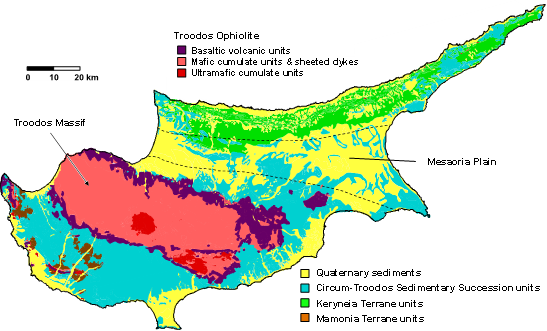# Concentration-concentration fractal modelling: a novel insight for correlation between variables in response to changes in the underlying controlling geological-geochemical processes

Behnam Sadeghi has recently published a research paper in “Ore Geology Reviews”. This paper is based on one of the methods Behnam has developed in his PhD thesis. It is about a quite novel fractal model, named Concentration-Concentration (C-C), applied to geochemical anomaly classification. Also, in this paper Behnam has proposed a Bayesian framework, using Monte Carlo simulation, to evaluate if the sampling has been done well enough statistically, in terms of the number and the quality of the samples collected. One more new idea in this paper is a decision-making method in a Bayesian framework to compare various classification methods based on two statistical parameters of uncertainty (risk) and return, using Monte Carlo simulation. It helps with comparing the robustness of the models per class, defined by thresholds. The C-C model is highly recommended to apply to exploration, urban and environmental geochemical studies.ABSTRACT:

Fractal/multifractal classification models applied to regional geochemical data are typically based on relationships between geochemical concentrations and mathematical, statistical or geometrical properties such as sample numbers, surface areas, perimeter and distance. There is, however, another component of geochemical datasets that is implicitly addressed using multi-variate methods such as factor analysis or various forms of clustering. This is the degree of correlation and changes in the nature of the correlation between elements as the processes affecting sample geochemistry change. In regional datasets, much of the change is related to differences in parent lithology or regolith type, but also relates to the effects of mineralisation or contamination. Where two elements display systematic changes in their mutual correlation in response to different ‘forcings’, joint modelling of such bivariate relationships can provide new ‘dimensions’ to data interpretation. Bivariate probability plots indicate distinct population breaks representing the intersections between different sub-populations within the two variables. This concept is extended in this study to fractal analysis and a proposed concentration-concentration (C–C) fractal model. A soil dataset from Cyprus was studied in this research to demonstrate and interpret the C–C results associated with copper (Cu)-related VMS mineralisation considering the geological-geochemical evidence. The C–C models generated based on the relation between Cu and indium (In) were compared to the established number-size (N-S), concentration-area (C-A), spectrum-area (S-A) and singularity models. All the models were applied to the same datasets, but in different formats, i.e., raw data, and multi-variate approaches of Box-Cox transformed data, factor analysis (FA) and centred log-ratio (clr) transformed data scores. This is to visualise and evaluate the robustness of the C–C model comparing to uni- and multi-variate studies. Therefore, based on the maps classified by the C–C model and the geology in Cyprus comparing to the mentioned models, the robustness of the C–C fractal model was demonstrated. In addition, applying Monte Carlo simulation on each class characterised by all the models applied, demonstrated a remarkably high certainty of the thresholds obtained by the C–C fractal model.

Full link to text is here.